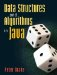# Amortized Analysis

### Projects

 7.19 Complete Figure 7-22. 7.2 Complete Figure 7-23. Drop any fractions and approximate very large values using scientific notation as shown. You will probably have to use both a calculator/computer and some algebra to accomplish this.

Figure 7-22. Comparison of algorithms from our ArrayList and LinkedList classes, for Project 7.19. (Analyze the version of remove() which takes an index as an argument.)

ArrayList

Best

Avg

Amort

Worst

Best

Avg

Amort

Worst

Q(1)

Q(n)

contains()

Q(1)

Q(n)

Q(n)

get()

Q(1)

Q(n)

isEmpty()

iterator()

remove()

size()

Q(n)

Q(n)

Q(n)

Q(n)

Figure 7-23. Number of elements that can be processed in a given amount of time for various running-time functions. For Project 7.20.

Time to process n elements (milliseconds)

1 second

1 minute

1 hour

1 day

1 year

log2 n

1018,061

n

1,000

60,000

3.1 x 1010

n log2 n

n2

1,897

n3

2n

9Data Structures and Algorithms in Java
ISBN: 0131469142
EAN: 2147483647
Year: 2004
Pages: 216
Authors: Peter Drake